# Finite group scheme

(diff) ← Older revision | Latest revision (diff) | Newer revision → (diff)

A group scheme that is finite and flat over the ground scheme. Ifis a finite group scheme over a scheme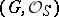, then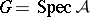, whereis a finite flat quasi-coherent sheaf of algebras over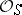. From now on it is assumed thatis locally Noetherian. In this caseis locally free. Ifis connected, then the rank of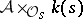over the field of residuesat a point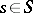is independent ofand is called the rank of the finite group scheme. Letbe the morphism of-schemes mapping an element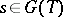into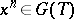, whereis an arbitrary-scheme. The morphism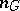is null if the rank ofdividesand ifis a reduced scheme or ifis a commutative finite group scheme (see Commutative group scheme). Every finite group scheme of rank, whereis a prime number, is commutative .

Ifis a subgroup of a finite group scheme, then one can form the finite group scheme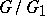, and the rank ofis the product of the ranks of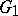and.

## Contents

### Examples.

1) Let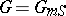be a multiplicative group scheme (or Abelian schemeover); thenis a finite group scheme of rank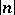(or).

2) Letbe a scheme over the prime field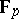and let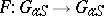be the Frobenius homomorphism of the additive group scheme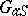. Then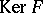is a finite group scheme of rank.

3) For every abstract finite group schemeof orderthe constant group schemeis a finite group scheme of rank.

The classification of finite group schemes over arbitrary ground schemes has been achieved in the case where the rank ofis a prime number (cf. ). The case whereis a commutative finite group scheme andis the spectrum of a field of characteristicis well known (see , , ).

How to Cite This Entry:
Finite group scheme. Encyclopedia of Mathematics. URL: http://encyclopediaofmath.org/index.php?title=Finite_group_scheme&oldid=15344
This article was adapted from an original article by I.V. Dolgachev (originator), which appeared in Encyclopedia of Mathematics - ISBN 1402006098. See original article